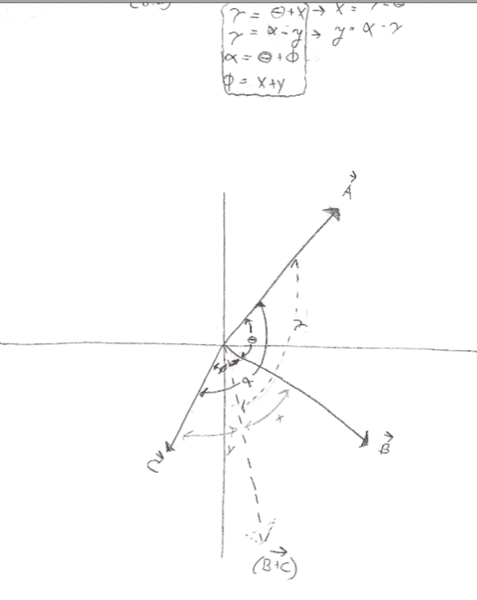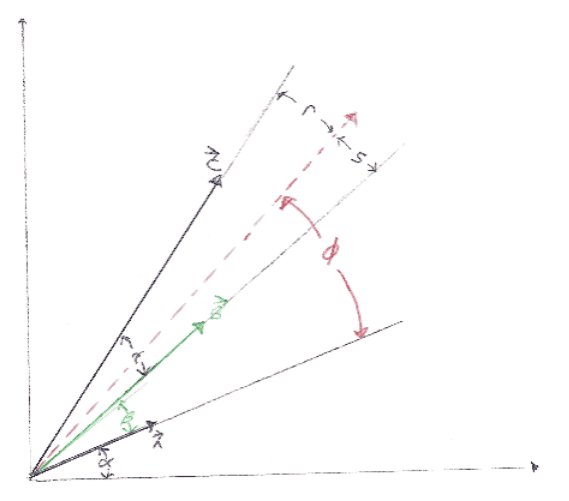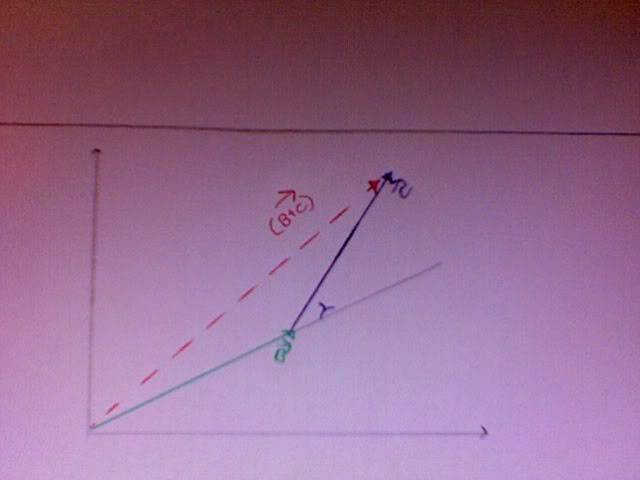# Show that Dot-Product is Distributive

## Homework Statement

Griffith's E&M problem 1.1. I feel good about my life.

Using the definition $\vec{A}\cdot\vec{B} =AB\cos\theta$ show that the dot product is distributive when

(a) the 3 vectors are coplanar
(b)the general case

Okay then for part (a) I have started like this:

Let A B & C be 3 coplanar vectors. Let $\theta$ be the angle between A & B; let $\phi$ be between B & C and let $\alpha$ be between A & C

then

$$\vec{A}\cdot(\vec{B}+\vec{C})=|\vec{A}||\vec{B+C}|\cos\gamma$$

where gamma is the angle between A and (B+C)

...now I am a little confused, i want to write that this implies

$$\vec{A}\cdot(\vec{B}+\vec{C})=(AB+AC)\cos\gamma$$

but I am not sure if that is correct. And if it is, where to go from here?

Last edited:

I also drew this and came up with the four relationships seen in the box. I am not sure if this helps meLet $$\vec{A} = (a_x,a_y)$$ etc. What is $$\vec{A} \cdot \vec{B}$$ in terms of $$a_x, a_y, b_x$$ and $$b_y$$?

I thought of using components, but the problem specifically asks to use the definition provided.

$\vec{A}\cdot\vec{B} =AB\cos\theta$

Well the intermediate step would rely on the definition. Plus it's easy to generalize.

Let $$\vec{A} = (a_x,a_y)$$ etc. What is $$\vec{A} \cdot \vec{B}$$ in terms of $$a_x, a_y, b_x$$ and $$b_y$$?

$$\vec{A} \cdot \vec{B}=(a_xb_x+a_yb_y)$$

$$\Rightarrow (\vec{A} \cdot \vec{B})\cdot\vec{C}=(a_xb_xc_x+a_yb_yc_x)$$

Right?

Not sure what this intermediate step is? I could do the same for $\vec{A} \cdot (\vec{B}\cdot\vec{C})$ and show that they are equal w/out using the definition

$$(\vec{A} \cdot \vec{B})\cdot\vec{C}$$

Don't you mean $$(\vec{A} + \vec{B})\cdot\vec{C}$$?Last edited:
Not sure what this intermediate step is? I could do the same for $\vec{A} \cdot (\vec{B}\cdot\vec{C})$ and show that they are equal w/out using the definition

The intermediate step is to say that $$\vec{A} \cdot \vec{B}=(a_xb_x+a_yb_y)$$ which is easier to work with in this case. You don't need to say $\vec{A} \cdot (\vec{B}\cdot\vec{C})$ is equal to anything and in fact you can't because the dot product is only for vectors.

Don't you mean $$(\vec{A} + \vec{B})\cdot\vec{C}$$?Sorry, I was working on another problem at the same timeOne minute....

okay...I still don't see how this:

$$(\vec{A} + \vec{B})\cdot\vec{C}=(a_x+b_x)c_x+(a_y+b_y)c_y=a_xc_x+b_xc_x+a_yc_y+b_yc_y=\vec{A}\cdot\vec{B}+\vec{B}\cdot\vec{C}$$

uses the definition of dot-product $\vec{A}\cdot\vec{B} =AB\cos\theta$

SorryTo prove that

$$\vec{A}\cdot\vec{B}=a_xb_x+a_yb_y$$

you have to use the definition. If the angle of $$\vec{A}$$ is $$\alpha$$ and the angle of $$\vec{B}$$ is $$\beta$$ then

$$\vec{A}\cdot\vec{B} = |\vec{A}||\vec{B}|\cos{(\alpha-\beta)}$$

$$=|\vec{A}||\vec{B}|(\cos{\alpha}\cos{\beta}+sin{\alpha}\sin{\beta})$$

$$=|\vec{A}|\cos{\alpha}|\vec{B}|\cos\beta+|\vec{A}|sin{\alpha}|\vec{B}|\sin{\beta}$$

$$=a_xb_x+a_yb_y$$

This is an interesting approach. Thanks qntty!

The only thing is that the question is asked (in the text) before the component-wise definition of the Dot-Product is even given.

This leads me to wonder if there is a way to show that the dot-product is distributive without ever going into components.

I think that my original approach might lead somewhere. You have given me an idea to modify it though. I like how you used an absolute reference frame when naming your angles, instead of the way I named them (i.e., wrt the vectors themselves).

I will play around with this a little, but it is really difficult for me to see a way to do this w/out components coming into play.

Thanks againOkay, scratch that last post. I am going to stick with my original way of labeling angles (i.e., wrt the vectors themselves, not the reference frame)

Here is my new diagram:Now, since I already know that the dot product distributes then I know that I am shooting for

$$(\vec{A} + \vec{B})\cdot\vec{C}=\vec{A}\cdot\vec{B}+\vec{ B}\cdot\vec{C}$$

So the end result should be

something=$|A||B|\cos\beta+|B||C|\cos\gamma$

From my diagram I have

$$(\vec{A} + \vec{B})\cdot\vec{C}=|A||B+C|\cos\phi$$

This leads me to believe that I must write $\phi$ in terms of $\beta$ and $\gamma$

From my diagram, I also have the relationships:

$\phi=\beta+s$
$\phi=(\beta+\gamma)-r$

Beta and gamma are 'known' and r, s, and phi are unknown....

Seems like I am one equation short of doing something.Any ideas?

Last edited:
gabbagabbahey
Homework Helper
Gold Member
Hint: $|\vec{B}+\vec{C}|=$___?

Hint: $|\vec{B}+\vec{C}|=$___?

I don't know without using componentsgabbagabbahey
Homework Helper
Gold Member
I don't know without using componentsTry drawing the tail of the vector C at the head of the vector B and draw in the vector B+C. Draw all the angles in and use the law of cosines.

Try drawing the tail of the vector C at the head of the vector B and draw in the vector B+C. Draw all the angles in and use the law of cosines.

If I do it this way, I think that I will need to go back to defining the angle wrt a datum instead of from vector to vector... other wise, i do not know what angles to write in.

gabbagabbahey
Homework Helper
Gold Member
Extend a dotted line along the direction of B and label $\gamma$ the same way as before...you should quickly see that one of the angles in your triangle is $\pi-\gamma$Hmmm. I don't see how an angle inside my triangle is pi-gamma.

Maybe I'm cooked ...

What if I drop a perpendicular from the tip of C to my extension of B.. then wouldn't the angle between B+C and C just be gamma?

gabbagabbahey
Homework Helper
Gold Member
Basic Trig....look at the large angle adjacent to gamma...if gamma were zero you would simply have a straight line (pointing in the direction of B) and that angle would be 180 degrees or pi radians, in general that angle is (180 deg- gamma)

gabbagabbahey
Homework Helper
Gold Member
What if I drop a perpendicular from the tip of C to my extension of B.. then wouldn't the angle between B+C and C just be gamma?

No, because B+C is not parallel to B

Last edited:
Basic Trig....look at the large angle adjacent to gamma...if gamma were zero you would simply have a straight line (pointing in the direction of B) and that angle would be 180 degrees or pi radians, in general that angle is (180 deg- gamma)

Oh manWhen I read your post, you said pi - gamma, but I was thinking pi/2 - gamma...pi = 180 ... my brain = pudding